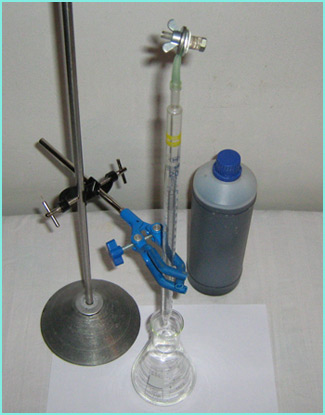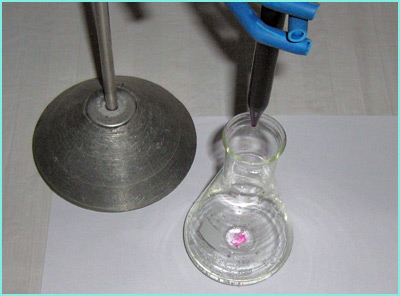Miscellaneous: Permanganatometry Permanganatometry is a method based on a redox reaction, which is often used in analytical chemistry for determining the concentration of various compounds. This method can also be used to determine the concentration of hydrogen peroxide in a given sample. The following equation applies: 5H2O2 + 2MnO4- + 6H+ -> 5O2 + 2Mn2+ + 8H2O One needs to prepare a burette, a solution of potassium permanganate and a sample containing hydrogen peroxide. In this case, an improvised burette based on a pipette has been used.The solution of potassium permanganate (0.02 mol/L) has been prepared by first dissolving 3.16 grams of the mentioned compound in a small amount of distilled water. Afterwards, more distilled water was added, until the volume reached the value of one liter. Since potassium permanganate is not a primary standard, in analytical laboratories usually standardization is done - this means that the real concentration is determined with the help of another reaction, i.e. titration, by using a primary standard (commonly sodium oxalate). In this case, that was not done, as a rough determination of the H2O2 was good enough for the purpose of this experiment, which was to document and show how a simple titration can be done. As for the solution of hydrogen peroxide, the sample has been prepared by adding 3.00 grams of the hydrogen peroxide solution to a 250 mL volumetric flask. Then more water has been added until the 250 mL mark was reached. Afterwards 25 mL of the prepared solution were transferred to another flask by using a pipette. To this solution, 10 mL of diluted sulphuric acid (1:5) have also been added. This sample was then titrated with the solution of potassium permanganate.At first, the potassium permanganate solution has been added a bit faster. However, with time, the addition has been slowed down, especially when it was noticed that the purple color doesn't disappear immidiately when in contact with the hydrogen peroxide solution anymore. From that moment on, the potassium permanganate solution has been added carefully, drop by drop, while gently swirling the flask. The titration has been discontinued at the moment when the purple (i.e. pink, due to the low concentration) color was present at least thirty to sixty seconds. Then the total volume of the potassium permanganate solution added to the hydrogen peroxide has been read from the burette and noted.In this case, 43.1 mL of potassium permanganate solution (c = 0.02 mol/L) has been used. By using the mentioned values, the amount of KMnO4 was easily calculated - it was equal to 8.62x10-4 moles. The reaction equation states that a certain amount of potassium permanganate reacts with 2.5x of hydrogen peroxide. Thereby, the amount of the hydrogen peroxide was 2.15x10-3 moles. This amount was contained in one tenth of the hydrogen peroxide solution that has been prepared in the volumetric flask. Thereby, 0.073 grams of H2O2 (2.15x10-3 moles) was present in 0.3 grams of the sample, which corresponds to a mass fraction of 24.4%.